Home > CC2MN > Chapter cc29 > Lesson cc29.1.3 > Problem9-45

9-45.
1.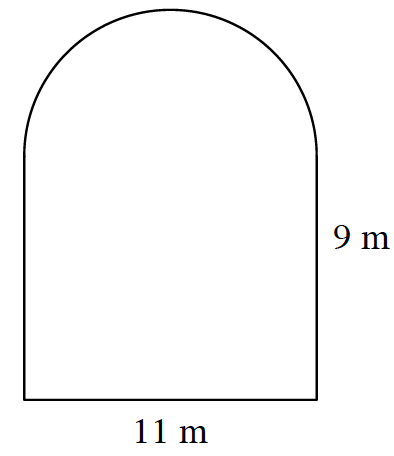The shape at right is composed of a rectangle and a semi‑circle (half of a circle). Homework Help ✎

1. Find the perimeter.

2. Find the area.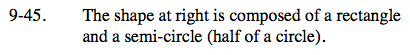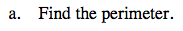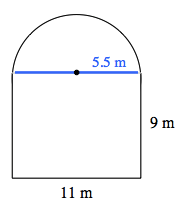The perimeter of a semi-circle is half of the circumference of a circle.

If the semi-circle were made into a complete circle, its circumference would be 11π.

46.27 meters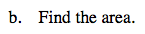Find the area of the semi-circle and the area of the rectangle separately, then add them together to find the total area.